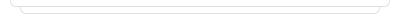Free Excel Training and CoursesLook at the some of richest people in the world using a table their age, earnings and their companies. And get informati...Look at the some of richest people in the world using a table their age, earnings and their companies. And get informati...### Practice: SUMIFS, COUNTIFS, AVERAGEIFS - II

Making more practice by using SUMIFS(), COUNTIFS() and AVERAGEIFS() functions.### Practice: SUMIFS, COUNTIFS, AVERAGEIFS - II

Making more practice by using SUMIFS(), COUNTIFS() and AVERAGEIFS() functions.### Practice: SUMIFS, COUNTIFS, AVERAGEIFS - V

Making more practice by using SUMIFS(), COUNTIFS() and AVERAGEIFS() functions.### Practice: SUMIFS, COUNTIFS, AVERAGEIFS - V

Making more practice by using SUMIFS(), COUNTIFS() and AVERAGEIFS() functions.### Practice: SUMIFS, COUNTIFS, AVERAGEIFS - I

Making more practice by using SUMIFS(), COUNTIFS() and AVERAGEIFS() functions.### Practice: SUMIFS, COUNTIFS, AVERAGEIFS - I

Making more practice by using SUMIFS(), COUNTIFS() and AVERAGEIFS() functions.### Practice: SUMIFS, COUNTIFS, AVERAGEIFS - IV

Making more practice by using SUMIFS(), COUNTIFS() and AVERAGEIFS() functions.### Practice: SUMIFS, COUNTIFS, AVERAGEIFS - IV

Making more practice by using SUMIFS(), COUNTIFS() and AVERAGEIFS() functions.### Practice: SUMIFS, COUNTIFS, AVERAGEIFS - III

Making more practice by using SUMIFS(), COUNTIFS() and AVERAGEIFS() functions.### Practice: SUMIFS, COUNTIFS, AVERAGEIFS - III

Making more practice by using SUMIFS(), COUNTIFS() and AVERAGEIFS() functions.Look at the some of richest people in the world using a table their age, earnings and their companies. And get informati...### Practice: SUMIFS, COUNTIFS, AVERAGEIFS - II

Making more practice by using SUMIFS(), COUNTIFS() and AVERAGEIFS() functions.### Practice: SUMIFS, COUNTIFS, AVERAGEIFS - V

Making more practice by using SUMIFS(), COUNTIFS() and AVERAGEIFS() functions.### Practice: SUMIFS, COUNTIFS, AVERAGEIFS - I

Making more practice by using SUMIFS(), COUNTIFS() and AVERAGEIFS() functions.### Practice: SUMIFS, COUNTIFS, AVERAGEIFS - IV

Making more practice by using SUMIFS(), COUNTIFS() and AVERAGEIFS() functions.### Practice: SUMIFS, COUNTIFS, AVERAGEIFS - III

Making more practice by using SUMIFS(), COUNTIFS() and AVERAGEIFS() functions.